# Modeling

The basic method for visualizing the results in our project is plate assay. According to this method, agar medium containing calcium phytate is poured onto Petri dishes. Then, a few wells are made in the medium and filled with yeast culture homogenate containing phytase. Phytase diffuses in the medium and breaks down calcium phytate. Agar then gets turbid as produced calcium phosphate precipitates (similar to enzyme immunoassay method). The results of such plate assay make it possible to assess protein’s enzymatic activity. But this method is viewed as not accurate enough though very demonstrative.

We decided to develop a model making this method of assessment more accurate. We believe that our model will make it possible to calculate the exact time required for the protein to diffuse in gel for a certain distance, thus eliminating the errors associated with the ‘overstaying’ of the plate and bias in assay results.

The developing of a detailed model including the analysis of Michaelis-Menten equation for enzymatic reaction is very complicated and requires additional elaboration; for this reason, for the purpose of our trial model we used approximation where concentration of calcium phytate in the medium is considered to be rather low and concentration of phytase is high enough, and therefore protein diffusion is accompanied by simultaneous substrate breakdown.

In our calculations, we used data from article (DOI: 10.1016/j.jconrel.2006.08.006). Besides, we need the hydrodynamic radius value of phytase. There is no such value for Obesumbacterium proteus phytase available in literature; therefore, we used the radius value for fungal phytase which is known (DOI: 10.1016/j.bbrc.2004.12.111). As these enzymes are similar in their structure, it can be supposed that such approximation will not influence the results in a significant way.

The hydrodynamic radius of active phytase equals to 4 nm.

Knowing this, we can calculate the diffusion coefficient for the protein in gel. For this purpose, we will first calculate its diffusion coefficient in water according to the following equation: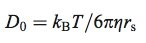- where kb is Boltzmann's constant, 1.38*10-23 J/K;

- Т is assay temperature (in our case its room temperature), 298 К;

- η is water viscosity coefficient, 0.6947 × 10−3 Pa*s;

- rs is hydrodynamic radius of the protein, 4 nm.

The calculated diffusion coefficient D0 for our protein is 7.85*10-7 cm2/s. The coefficient of diffusion in gel Dg relates to D0 according to the following equation: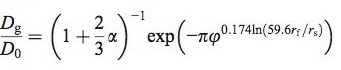- where rs is hydrodynamic radius of the protein;

- rf is radius of agarose fibers (according to DOI: 10.1016/j.jconrel.2006.08.006);

- and φ and α can be calculated using the following equations: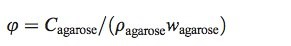The values of Ρ and ω are used as in article (DOI: 10.1016/j.jconrel.2006.08.006):

Ρ = 1.64 g/ml, ω = 0.625;

С = 0.018 g/ml;

then φ = 0.0176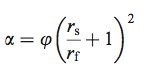- In our case α = 0.170.

- then Dg/D0 = 0.66. Dg = 0.66 * 7.85*10-7 = 5.22*10-7 cm2/c.

- The squared radius of diffusion is calculated as: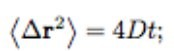For our model we will use the diffusion radius which is equal to 5 mm. We will enter the value of diffusion coefficient to the equation and get the value of diffusion time which is equal to 0.012 * 107 s= 33.3 hours, or 33 hours 20 minutes.

In order to build a more complicated model, diffusion of phytates in the medium from higher concentration regions to lower concentration regions should also be taken into consideration.

We can calculate the coefficient of phytate diffusion in gel as we know the coefficient of its diffusion in water (280 μm2 s-1). First, we will calculate the hydrodynamic radius according to the first equation. It will be:

rs = 1.12 nm

Now the alfa coefficient is equal to 0.044

Dg/D0 = 0.971 x 0.8847 = 0.86

Dg = 0.86 x 280 = 240.55 μm2 s-1

We will build a differential equation system that would describe the behavior of reaction components in gel. We will use the approximation where the changing of medium colour becomes visible when calcium phosphate concentration is increased hundredfold, that is when the concentration of calcium phytate is changed hundredfold.

The system of partial differential equations: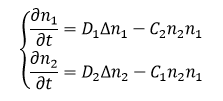Where n_1 is protein concentration measured in mol/cm3;

n2 is phytine concentration measured in mol/cm3;

D1 is coefficient of protein diffusion in gel measured in cm2/s;

D2 is coefficient of phytine diffusion in gel measured in cm2/s.

C1, is the protein-phytine biomolecular reaction rate constant which is equal to moles of phytine destroyed by the protein in 1 cm3 when protein and phytine concentration is 1 mol/cm3. The biomolecular reaction constant has the dimension of cm3mol-1s-1

We will use r as diffusion distance from the well center to a protein molecule.

• ∂n1/∂t describes the changing of the substance amount at point r over period t. The amount of substance at a given point r can change in two ways: 1) due to diffusion, which is described by D*nabla*n1, 2) reduction due to reaction with another substance, which is described by -C*n1*n2.

• The same is true for the second substance n2. However, as we neglect degradation of the protein (it has high stability at the given temperature and is not expended in reaction with phytine), then –C2*n1*n2 can be neglected as well.

If we put aside the influence of edge effects caused by finite height sizes of gel on the process of diffusion, then concentration of the substances will ultimately depend only on the distance to the center of the well where the protein is. We will identify this distance as r and move to the cylindrical coordinate system; then the Laplace operator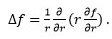The total is: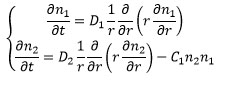where n1, n2 depend only on r, t.

To solve this system, initial condition are required: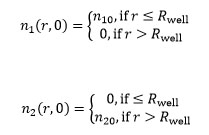The task parameters have been taken as follows: Rwell=0.25 cm , D1=5.22*10-7 cm2/s , D2=24.055*10-7 cm2/s, C1=8*103 cm3mol-1s-1, n10=5.53*10-8 mol/cm3, n20=3.03*10-8 mol/cm3. (The reaction rate constant value has been calculated based on the protein activity value = 9.6 µmol/(min*mg); the approximate protein concentration in homogenate was taken as 2.5 mg/ml – the approximation based on empirical experience; the data are taken from “Gene cloning, expression and characterization of novel phytase from Obesumbacterium proteus”, FEMS Microbiology Letters 236 (2004) 283–290; the activity value was taken for cell homogenate before protein purification).

1 second in the video = 10 days

The dynamic changing of protein concentration in gel and in the well has been calculated.

Obtained experimental data agrees quite well with calculated values